### Home > AC > Chapter 12 > Lesson 12.2.2 > Problem12-50

12-50.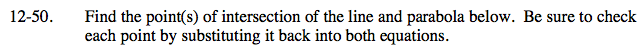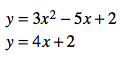Set the equations equal to each other.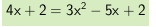Set the equation equal to zero.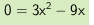Factor and Solve.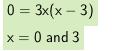Check these answers by substituting them back into the original equations.

Points of intersection are (3, 14) and (0, 2).